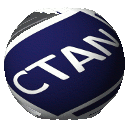# Directory graphics/pgf/contrib/tikz-feynman

TikZ-Feynman=============================================================

TikZ-Feynman is a package allowing Feynman diagrams to be easily generated within with minimal user instructions and without the need of external programs. It builds upon the TikZ package and leverages the graph placement algorithms from TikZ in order to automate the placement of many vertices. TikZ-Feynman still allows fine-tuned placement of vertices so that even complex diagrams can still be generated with ease.

TikZ-Feynman is made available through the Comprehensive Archive Network (CTAN) and comes with some thorough documentation containing a tutorial and many examples. Please refer to the project page for additional information.

TikZ-Feynman is open source and contribution are welcome. If you have any suggestions, feature requests, or have found any bugs, feel free to create a new issue or pull request on Github.

Below are a few example to demonstrate how easy diagram can be, and how extensible it can be. Many more are given in the documentation:

\feynmandiagram [horizontal=a to b] {
i1 -- [fermion] a -- [fermion] i2,
a -- [photon] b,
f1 -- [fermion] b -- [fermion] f2,
};


\feynmandiagram [large, vertical=e to f] {
a -- [fermion] b -- [photon, momentum=$$k$$] c -- [fermion] d,
b -- [fermion, momentum'=$$p_{1}$$] e -- [fermion, momentum'=$$p_{2}$$] c,
e -- [gluon]  f,
h -- [fermion] f -- [fermion] i;
};


\begin{tikzpicture}
\begin{feynman}
\vertex (a1) {$$\overline b$$};
\vertex[right=1cm of a1] (a2);
\vertex[right=1cm of a2] (a3);
\vertex[right=1cm of a3] (a4) {$$b$$};
\vertex[right=1cm of a4] (a5);
\vertex[right=2cm of a5] (a6) {$$u$$};

\vertex[below=2em of a1] (b1) {$$d$$};
\vertex[right=1cm of b1] (b2);
\vertex[right=1cm of b2] (b3);
\vertex[right=1cm of b3] (b4) {$$\overline d$$};
\vertex[below=2em of a6] (b5) {$$\overline d$$};

\vertex[above=of a6] (c1) {$$\overline u$$};
\vertex[above=2em of c1] (c3) {$$d$$};
\vertex at ($(c1)!0.5!(c3) - (1cm, 0)$) (c2);

\diagram* {
{[edges=fermion]
(b1) -- (b2) -- (a2) -- (a1),
(b5) -- (b4) -- (b3) -- (a3) -- (a4) -- (a5) -- (a6),
},
(a2) -- [boson, edge label=$$W$$] (a3),
(b2) -- [boson, edge label'=$$W$$] (b3),

(c1) -- [fermion, out=180, in=-45] (c2) -- [fermion, out=45, in=180] (c3),
(a5) -- [boson, bend left, edge label=$$W^{-}$$] (c2),
};

\draw [decoration={brace}, decorate] (b1.south west) -- (a1.north west)
node [pos=0.5, left] {$$B^{0}$$};
\draw [decoration={brace}, decorate] (c3.north east) -- (c1.south east)
node [pos=0.5, right] {$$\pi^{-}$$};
\draw [decoration={brace}, decorate] (a6.north east) -- (b5.south east)
node [pos=0.5, right] {$$\pi^{+}$$};
\end{feynman}
\end{tikzpicture}


## Licence

TikZ-Feynman

Feynman Diagrams with TikZ

This documentation may be redistributed and/or modified under the terms of the GNU General Public License as published by the Free Software Foundation, either version 3 of the License, or (at your option) any later version.

The code of this package may be distributed and/or modified under the conditions of the Project Public License, either version 1.3 of this license or (at your option) any later version.

This work has the LPPL maintenance status maintained'. The Current Maintainer of this work is Joshua Ellis.

This package is distributed in the hope that it will be useful, but WITHOUT ANY WARRANTY; without even the implied warranty of MERCHANTABILITY or FITNESS FOR A PARTICULAR PURPOSE.